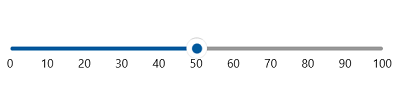# Getting Started with WinUI Slider

9 Aug 20213 minutes to read

This section explains the steps required to add the WinUI Slider control and its elements such as values, ticks, dividers, labels, and tooltip. This section covers only basic features needed to get started with Syncfusion Slider.

## Creating an application with WinUI Slider

1. Add reference to Syncfusion.Sliders.WinUI NuGet.

2. Import the control namespace `Syncfusion.UI.Xaml.Sliders` in XAML or C# code.

3. Initialize the `SfSlider` control

The default value of the `Minimum` and `Maximum` properties of the `SfSlider` is 0 and 100 respectively. So, the `Value` property must be given within the range.

``<slider:SfSlider />``
``````SfSlider sfSlider = new SfSlider();
this.Content = sfSlider;``````

## Set Value

You can show value in the slider by setting double value to the `Value` properties.

``<slider:SfSlider Value="50" />``
``````SfSlider sfSlider = new SfSlider();
sfSlider.Value = 50;
this.Content = sfSlider;``````## Enable Ticks

You can enable ticks in the slider using the `ShowTicks` property.

``````<slider:SfSlider Value="50"
ShowTicks="True" />``````
``````SfSlider sfSlider = new SfSlider();
sfSlider.Value = 50;
sfSlider.ShowTicks = true;
this.Content = sfSlider;``````## Enable Labels

You can enable labels in the slider using the `ShowLabels` property.

``````<slider:SfSlider Value="50"
ShowLabels="True" />``````
``````SfSlider sfSlider = new SfSlider();
sfSlider.Value = 50;
sfSlider.ShowLabels = true;
this.Content = sfSlider;``````## Enable Dividers

You can enable dividers in the slider using the `ShowDividers` property.

``````<slider:SfSlider Value="50"
ShowDividers="True"
DividerHeight="4"
DividerWidth="4"
ActiveTrackHeight="4"
InactiveTrackHeight="4" />``````
``````SfSlider sfSlider = new SfSlider();
sfSlider.Value = 50;
sfSlider.ShowDividers = true;
sfSlider.DividerHeight = 4;
sfSlider.DividerWidth = 4;
sfSlider.ActiveTrackHeight = 4;
sfSlider.InactiveTrackHeight = 4;
this.Content = sfSlider;``````NOTE